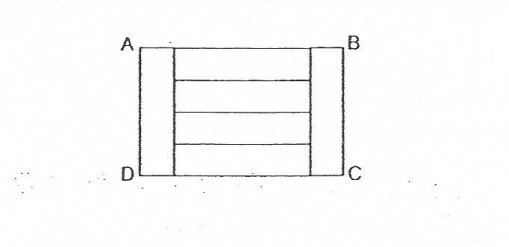# Question 1 of 52

Rectangle ABCD is divided into 6 identical small rectangle as shown below. Give that the perimeter of rectangle ABCD is 80cm, find the area of one small rectangle.A
64cm$^2$
B
68cm$^2$
C
80cm$^2$
D
72cm$^2$
E
None of the above